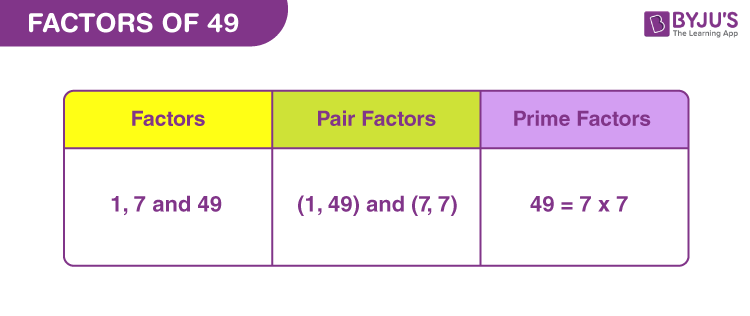# Factors Of 49

In Mathematics, factors of 49 are the real numbers that can divide the original number, evenly or in equal parts. Alternatively, we can say, when the product of two numbers results in the original number, then they become the factors. Since 49 is a composite number, it has more than two factors. We need to find these factors with the help of the division method. Also, the prime factors can be evaluated using the prime factorisation method.

Now, let us find the factors, prime factors and pair factors of 49 in this article.## How to Find Factors of 49?

Factors are the whole numbers that divide the original number (in this case, 49), completely.

• 49 ÷ 1 = 49
• 49 ÷ 7 = 7
• 49 ÷ 49 = 1

Thus, the factors of 49 are 1, 7 and 49.

## Pair Factors of 49

Pair factors or factors in pairs are the numbers that result in the original number (in this case 49) when multiplied together.

• 1 × 49 = 49
• 7 × 7 = 49

Therefore, the pair factors are (1, 49) and (11, 13).

Now, if we consider the negative pair factors, then;

• -1 × -49 = 49
• -7 × -7 = 49

Therefore, the negative pair factors are  (-1, -49) and (-7, -7).

## Prime Factorisation of 49

Prime factors of 49 are the prime numbers that divide the original number, completely. The list of prime numbers from 1 to 50 is given below to figure out the prime factors.

List of prime numbers (1 to 50) :

 2, 3, 5, 7, 11, 13, 17, 19, 23, 29, 31, 37, 41, 43, 47

Step 1:
From the above, 49 is completely divisible by 7. Thus, the smallest prime factor for 49 is 7.

49/7 = 7

Step 2: 7 itself is a prime number, thus it is divisible by either 1 or itself.

7/7 = 1

Further division is not possible because we already have a dividend equal to 1.

Therefore,

 Prime factorisation of 49 = 7 x 7

## Video Lesson on Prime Factors## Solved Examples

Q.1: If 7 roses are bought for Rs.49. Then what is the cost of each rose?

Solution: Given,

Number of roses bought = 7

Cost of 7 roses = Rs. 49

Cost of each rose = 49/7 = 7

Therefore, each rose cost Rs. 7.

Q.2: Find the sum of all the factors of 49. Also find the average.

Solution: The factors of 49 are 1, 7 and 49.

Sum = 1 + 7 + 49 = 57

Therefore, 157 is the required sum.

Now, to find the average of the factors, we need to divide the sum by total number factors.

Hence,

Average = 157/3 = 52.33

Q.3: What is the greatest common factor of 45 and 49?

Answer: Let us write the factors of both the numbers.

49 → 1, 7, 49

45 → 1, 3, 5, 9, 15, 45

Hence, the greatest common factor is only 1.

Q.4: What is the GCF of 49 and 84?

Solution: We know the factors of 49 are 1, 7 and 49.

Factors of 84 = 1, 2, 3, 4, 6, 7, 12, 14, 21, 28, 42, 84

If we compare, 7 is the greatest common factor of 49 and 84.

GCF (49, 84) = 7

## Frequently Asked Questions – FAQs

### What are the factors of 49?

There are a total of three factors of 49. These factors are 1, 7 and 49.

### How is 49 represented as the product of prime factors?

The prime factorisation of 49 is:
49 = 7 x 7 = 72

### What are the multiples of 49?

The first 10 multiples of 49 are 49, 98, 147, 196, 245, 294, 343, 392, 441 and 490.

### Is 3 a factor of 49?

No, 3 is not a factor of 49. 3 cannot divide 49 completely.

### Is 49 a prime number?

No, 49 is a composite number because it has more than 2 factors.

### Is 49 a perfect square?

Yes, 49 is a perfect square because the root of 49 will result in a whole number.
√49 = 7

Test your knowledge on Factors of 49